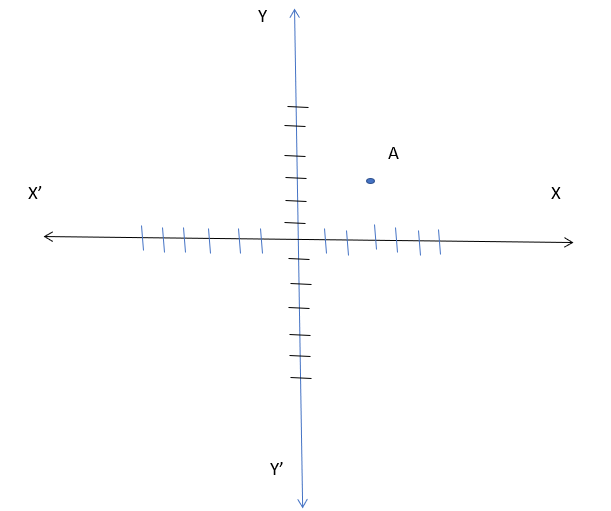Maths-
General
Easy

Question

# Write the coordinates of A:## (-3, 0)(0,3)(-3,-3)(3,3)Hint:

## The correct answer is: (3,3)

### A point in x-y plane has two coordinates x and y.x coordinate gives the distance of the point from y-axis.y coordinate gives the distance of the point from x-axis.From the figure we can see that, the distance of the point from y-axis is 3 units and the distance of the point from x-axis is 3 units.The coordinates of the points are (3,3)

For such questions, we have to count the distance of from both the axis#### With Turito Foundation.#### Get an Expert Advice From Turito.